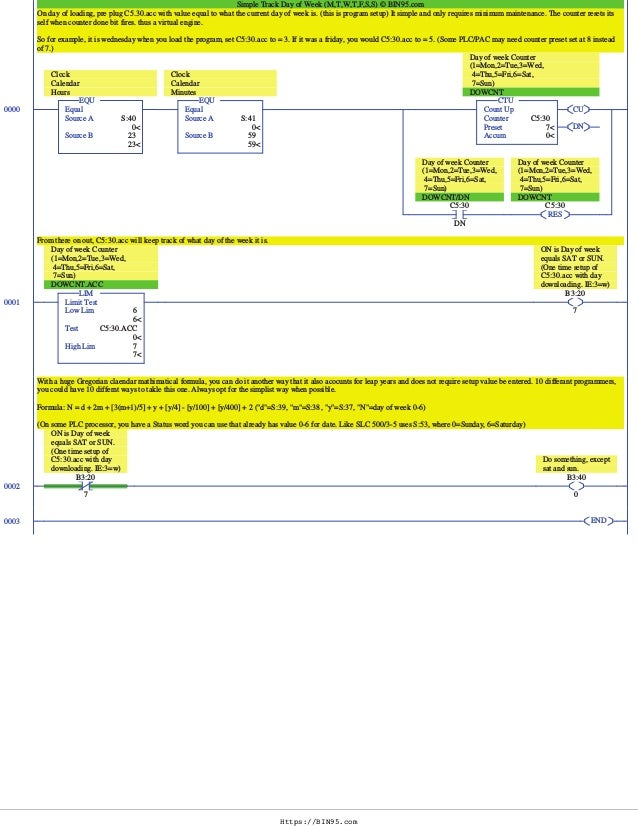Successfully reported this slideshow.
We use your LinkedIn profile and activity data to personalize ads and to show you more relevant ads. You can change your ad preferences anytime.Upcoming SlideShare
Loading in …5
×

# Plc programming example Day of Week - Answ

Answer to Day of Week PLC programming example we placed at https://www.slideshare.net/bin95/plc-programming-example-day-of-week to see if those starting out with PLC programming could figure out how to fix. The fix is in, this it.

This is a simple solution to lab manual exercise in downloadable PLC Training Certificate course at https://bin95.com/allen-bradley-plc-programming.htm Last rung comment the solution show here also gives 2 alternate solutions (newer plc solution, and complex formula solution.) A simple solution for tracking Day of week (Mon, Tues, etc.) part of HVAC scenario 3 (page 109 of built in Lab Manual for above course).

The version you are viewing here, PLC-Programing-Example-Day-of-Week.rss is one possible working solution for the Day-of-Week PLC (DOW) program.

Also if your PLC does not have a real time clock (RTC), you could use https://www.slideshare.net/bin95/plcclock in place of RTC status bits. In some PLCs, you could use a RTC instruction.

• Full Name
Comment goes here.

Are you sure you want to Yes No
Your message goes here• Login to see the comments

### Plc programming example Day of Week - Answ

1. 1. Https://BIN95.com Simple Track Day of Week (M,T,W,T,F,S,S) � BIN95.com On day of loading, pre plug C5.30.acc with value equal to what the current day of week is. (this is program setup) It simple and only requires minimum maintenance. The counter resets its self when counter done bit fires. thus a virtual engine. So for example, it is wednesday when you load the program, set C5:30.acc to = 3. If it was a friday, you would C5:30.acc to = 5. (Some PLC/PAC may need counter preset set at 8 instead of 7.) 0000 EQU Equal Source A S:40 0< Source B 23 23< EQU Clock Calendar Hours EQU Equal Source A S:41 0< Source B 59 59< EQU Clock Calendar Minutes CU DN CTU Count Up Counter C5:30 Preset 7< Accum 0< CTU Day of week Counter (1=Mon,2=Tue,3=Wed, 4=Thu,5=Fri,6=Sat, 7=Sun) DOWCNT C5:30 DN Day of week Counter (1=Mon,2=Tue,3=Wed, 4=Thu,5=Fri,6=Sat, 7=Sun) DOWCNT/DN RES C5:30 Day of week Counter (1=Mon,2=Tue,3=Wed, 4=Thu,5=Fri,6=Sat, 7=Sun) DOWCNT From there on out, C5:30.acc will keep track of what day of the week it is. 0001 LIM Limit Test Low Lim 6 6< Test C5:30.ACC 0< High Lim 7 7< LIM Day of week Counter (1=Mon,2=Tue,3=Wed, 4=Thu,5=Fri,6=Sat, 7=Sun) DOWCNT.ACC B3:20 7 ON is Day of week equals SAT or SUN. (One time setup of C5:30.acc with day downloading. IE:3=w) With a huge Gregorian claendar mathimatical formula, you can do it another way that it also acocunts for leap years and does not require setup value be entered. 10 differant programmers, you could have 10 differnt ways to takle this one. Always opt for the simplist way when possible. Formula: N = d + 2m + [3(m+1)/5] + y + [y/4] - [y/100] + [y/400] + 2 ("d"=S:39, "m"=S:38, "y"=S:37, "N"=day of week 0-6) (On some PLC processor, you have a Status word you can use that already has value 0-6 for date. Like SLC 500/3-5 uses S:53, where 0=Sunday, 6=Saturday) 0002 B3:20 7 ON is Day of week equals SAT or SUN. (One time setup of C5:30.acc with day downloading. IE:3=w) B3:40 0 Do something, except sat and sun. 0003 END